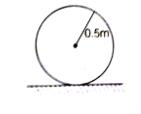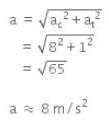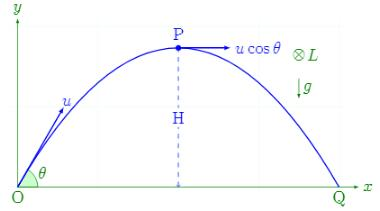Courses

# Test: Angular Momentum

## 10 Questions MCQ Test Physics Class 11 | Test: Angular Momentum

Description
This mock test of Test: Angular Momentum for JEE helps you for every JEE entrance exam. This contains 10 Multiple Choice Questions for JEE Test: Angular Momentum (mcq) to study with solutions a complete question bank. The solved questions answers in this Test: Angular Momentum quiz give you a good mix of easy questions and tough questions. JEE students definitely take this Test: Angular Momentum exercise for a better result in the exam. You can find other Test: Angular Momentum extra questions, long questions & short questions for JEE on EduRev as well by searching above.
QUESTION: 1

### A person standing on a rotating platform with his hands lowered outstretches his arms. The angular momentum of the person

Solution:

Yes because there is absence of any external force or torque so angular momentum will remain constant
here outstretching the hands means internal forces are working.. so moment of inertia increases in this case and to make angular momentum constant when angular velocity decreases.

QUESTION: 2

### The mass of an electron is  9 x 10-31 kg. It revolves around the nucleus of an atom in a circular orbit of radius 4.5 Å, with a speed of  8 x 105m/s. The angular momentum of electron is

Solution:

M=9x10-31Kg,r=4.5x10-10
V=8x105m/s
L=mvr
=9x10-31 x 4.5 x 10-10 x 8 x 10= 3.24 x 10-34 kgm2s-1

QUESTION: 3

### The earth rotates about an axis passing through its north and south poles with a period of one day. If it is struck by meteorites, then

Solution:

Because angular momentum remains constant as the moment of inertia is increasing here so the angular velocity will decrease.

QUESTION: 4

Relation between torque and angular momentum is similar to the relation between

Solution:

Torque is the rate of change of angular momentum. Force in linear motion corresponds to torque in rotary motion. Thus, just as Newton’s second law can be written as F = dP/dt (F for linear motion), it can be written as T = dL/dt for rotary motion.

QUESTION: 5

The dimensions of angular momentum are

Solution:

We know that angular momentum,
L = r x P
Thus its dimension is [r][P] = ML2T-1.

QUESTION: 6

An earth satellite is moving around the earth in a circular orbit. In such case, what is conserved?

Solution:

An earth satellite is moving in a circular motion due to change in the direction of velocity. Its angular momentum about centre is conserved as there is no external force acting on the system.

QUESTION: 7

A uniform circular disc of radius 50 cm at rest is free to turn about an axis which is perpendicular to its plane and passes through its centre. It is subjected  to a torque which produces a constant angular acceleration of 2.0 rad/sec2. It's net acceleration in m/s2 at the end of 2.0 s is a approximately.

Solution:

A uniform circular disc of radius 50 cm at rest is free to turn about an axis having perpendicular to its plane and passes through its centre. This situation can be shown by the figure given below:Therefore,

Angular acceleration = α = 2 rad/s2

Angular speed, ω = αt = 4 rad/s

Centripetal acceleration, ac = ω2r

42 x 0.5

=16 x 0.5

= 8m/s2

Linear acceleration at the end of 2 s is,

at = αt = 2 x 0.5 = 1 m/s2

Therefore, the net acceleration at the end of 2.0 sec is given byQUESTION: 8

kgm2s-1 is the unit of

Solution:

L = Iw
L is the angular momentum, I is the MOI and w is the rotational velocity.
[I] = [ML2T0], [w] = [M0L0T-1]
[L] = [MLT-1]

QUESTION: 9

Angular momentum is equal to____.(All the symbols used have their usual meanings)

Solution:

Angular momentum is equal to L = Iω. It is the formula.

QUESTION: 10

In case of a projectile, the angular momentum is minimum

Solution:In case of a projectile, the angular momentum is minimum at the starting point.